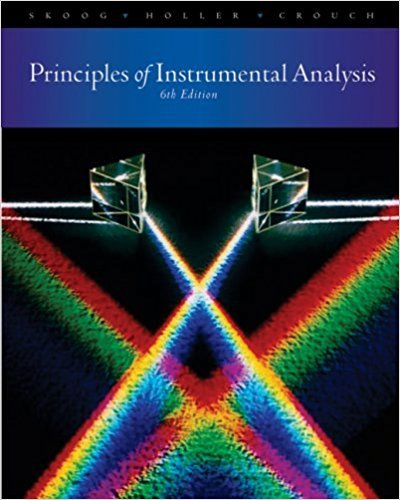×
×

# The maximum percentage error of a voltage processed by an ADC is given by thefollowingISBN: 9780495012016 317

## Solution for problem 4-10 Chapter 4

Principles of Instrumental Analysis | 6th Edition

• Textbook Solutions
• 2901 Step-by-step solutions solved by professors and subject experts
• Get 24/7 help from StudySoup virtual teaching assistantsPrinciples of Instrumental Analysis | 6th Edition

4 5 1 329 Reviews
14
5
Problem 4-10

The maximum percentage error of a voltage processed by an ADC is given by thefollowing equation:If the same ADC is used, how do the percentage errors in measured voltagescompare if the measured voltages arc 10 V and 1 V"

Step-by-Step Solution:
Step 1 of 3

Chem 107­Chapter 1, Measuring Matter and Energy What is Chemistry 1. Chemistry is the study of matter: a. Matter is anything that has mass and takes up space (volume). b. Energy has no mass and takes up no space. i. The ability of something to cause change or to do work. c. A substance (or chemical) is a particular...

Step 2 of 3

Step 3 of 3

##### ISBN: 9780495012016

Unlock Textbook Solution